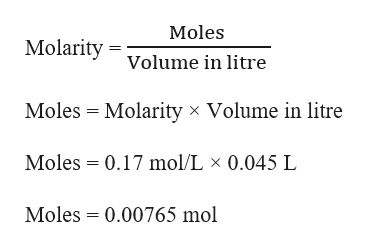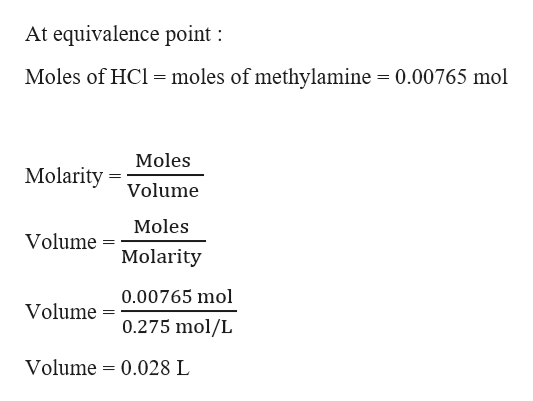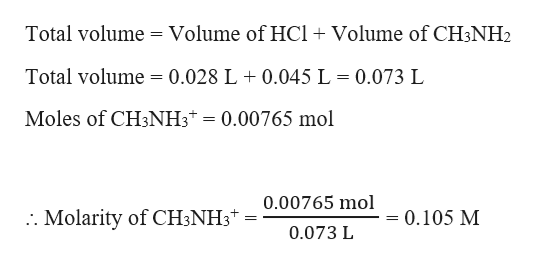# Calculate the pH at the equivalence point in the titration of 45.0 mL of 0.170 M methylamine(Kb = 4.4×10−4)with 0.275 M HCl.

Question
1 views

Calculate the pH at the equivalence point in the titration of 45.0 mL of 0.170 M methylamine

(
Kb = 4.4
×
10−4
)

with 0.275 M HCl.

check_circle

Step 1

Given,

Volume of methylamine = 0.170 M = 0.17 mol/L

Molarity of methylamine = 45.0 mL = 0.045 L     (1 mL = 0.001 L)

Molarity of HCl = 0.275 M = 0.275 mol/L

Moles of methylamine can be calculated as :help_outlineImage TranscriptioncloseMoles Molarity olume in litre Moles Molarity x Volume in litre Moles 0.17 mol/L x 0.045 L Moles 0.00765 mol fullscreen
Step 2

The reaction of methylamine with strong acid, HCl can be written as :

CH3NH2 (aq) + H+ (aq) → CH3NH3+ (aq)

At equivalence point, the moles of methyl amine will be equal to the moles of HCl. Thus, we can calculate the volume of HCl used as:help_outlineImage TranscriptioncloseAt equivalence point Moles of HCl moles of methylamine 0.00765 mol Moles Molarity Volume Moles Volume Molarity 0.00765 mol Volume 0.275 mol/L Volume 0.028 L fullscreen
Step 3

At equivalence point, the concentration of CH3NH3+ ...help_outlineImage TranscriptioncloseTotal volume = Volume of HCl Volume of CH3NH2 Total volume 0.028 L 0.045 L = 0.073 L Moles of CH3NH3 = 0.00765 mol 0.00765 mol Molarity of CH3NH3 = 0.105 M 0.073 L fullscreen

### Want to see the full answer?

See Solution

#### Want to see this answer and more?

Solutions are written by subject experts who are available 24/7. Questions are typically answered within 1 hour.*

See Solution
*Response times may vary by subject and question.
Tagged in

### Chemistry